ConicBundle
abstract positive semidefinite cone oracle

for the minimization of the maximum eigenvalue of affine matrix functions (see also implemention of a PSCOracle (PSCAffineFunction)) or, equivalently, Lagrangian relaxation of Linear Programs over the cone of positive semidefinite matrices More...

## Classes

class  ConicBundle::PSCPrimalExtender
Interface for extending PrimalData, e.g., in Lagrangian relaxation of column generation approaches. More...

class  ConicBundle::PSCOracle
Oracle interface for minimization of the maximum eigenvalue of an affine matrix function or, equivalently, Lagrangian relaxation of semidefinite programs. More...

class  ConicBundle::PSCBundleParameters
Bundle parameters for PSCModel, we recommend no to modify them. More...

## Detailed Description

for the minimization of the maximum eigenvalue of affine matrix functions (see also implemention of a PSCOracle (PSCAffineFunction)) or, equivalently, Lagrangian relaxation of Linear Programs over the cone of positive semidefinite matrices

The oracle for the positive semidefinite cone is based on the observation, that the support function over the positive semidefinite matrices with trace 1 is the maximum eigenvalue function,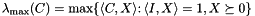For (explicitly or implicitly given) symmetric matrices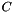and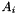of order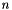for, the abstract PSCOracle represents one of the following convex functions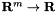depending on the selected parameter ConicBundle::FunctionTask and the scalar factor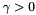when adding the function to the problem by MatrixCBsolver::add_function(),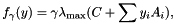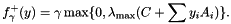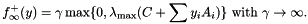While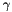is the fixed value given explicitly in MatrixCBsolver::add_function() in the first two cases, in the last case only the initial value is supplied and then solver and model successively determine new values so as to ensure (if feasible) that in the end solutions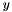(almost) satisfy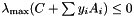.

The solver exploits a PSCOracle by using a specialized PSCModel which implements the cutting model of the spectral bundle method, i.e., the cutting surface is determined by an approximation of the active eigenspace of the maximum eigenvalue successively constructed by requesting maximum eigenvalue/eigenvector evaluations via PSCOracle::evaluate(). The approach is explicitly intended for large scale matrices, therefore PSCOracle never supplies the coefficient matrices explicitly but provides access to computing their action on given vectors or matrices and to the evaluation of the mamximum eigenvalue/eigenvector for given. In this sense it is a matrix free oracle. For an implementation that supports various special kinds of matrices (e.g. sparse and low rank matrices) see implemention of a PSCOracle (PSCAffineFunction). Of course special situations may allow for significantly more efficient eigenvalue/eigenvector computations than the general purpose iterative Lanczos method included there. In this case it is recommended to implement a corresponding specialized class derived from PSCOracle.

If in setting up groundset within MatrixCBSolver thevariables are introduced with a linear cost vector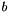and appropriate sign constraints, the three functions above may be interpreted as the Lagrangian dual to a primal semidefinite program of the form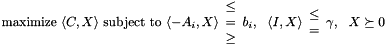The constraint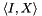on the trace is always added implicitly and its type is guided by the choice of ConicBundle::FunctionTask in MatrixCBSolver::add_function(). In particular

• FunctionTask::ObjectiveFunction represents the constraint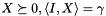. Lagrangian relaxation of the constraints on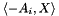by multipliers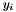then results in the dual function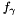. It corresponds to a primal feasible set having constant traceNote, if the other constraints already imply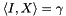, the dual solutionwill not be unique, because adding a constant to the diagonal of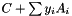will give rise to the same objective value.
• FunctionTask::ConstantPenaltyFunction represents the constraint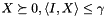. Lagrangian relaxation of the constraints onby multipliersresults in the dual function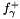. It corresponds to a primal feasible set having bounded trace. If a bound on the trace of the primal optimal solution is known in advance, this is a good choice for, and this variant is then more suitable than the next and last one.
• FunctionTask::AdaptivePenaltyFunction represents the constraintfor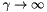, so in fact only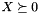. Lagrangian relaxation of the constraints onby multipliersresults in the dual function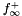. The given initial value ofwill be increased by PSCModel whenever there is no progress in forcing the maximum eigenvalue of the affine matrix function to a value of at most 0. If the primal problem has a bounded optimal solution, this will end at some point, butmight get too large for keeping numerical stability. In general this option is still a bit hazardous, the other two variants are clearly to be preferred. In particular, if a good bound on reasonable sizes of optimal solutions are known, it is suggested to choose ConstantPenaltyFunction instead.

In terms of the primal SDP view the sense of the constraints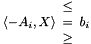is determined by the sign constraints on the dual variablesand these must be encoded in the ConicBundle::Groundset of the y variables, these bounds and the right hand side are not provided by this oracle. The right hand side can be added e.g. as a linear cost term for the variablesor alternatively via an AffineFunctionTransformation.

It is convenient to summarize the effect of theby introducing the mapping (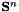refers to the set of real symmetric matrices of order)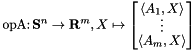The name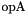or of its adjoint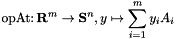will appear frequently in the names of the members of PSCOracle and its derived classes.

The bundle method computes the cutting model by successively computing and collecting eingenvectors to the maximum eigenvalue of the maximum eigenvalue function. The proper convex/conic combination of these, not needed explicitly yet implicitly computed by the method, successively approximates an optimal solution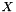to the primal SDP. As this primal approximation is often the central object of interest (e.g. it is the basis for cutting plane approaches) the method can be instructed to collect this primal information explicitly by supplying some PSCPrimal (similar to MatrixPrimal in MatrixFunctionOracle) in the implementation of PSCOracle::generate_minorant(). As the bundle method is designed for large scale matrices, collecting the full matrices (implemented in DensePSCPrimal) might be computationally too expensive, but other variants like collecting only the entries on some sparse support (e.g. in SparsePSCPrimal), sparse combined with a low rank dense part (e.g. in GramSparsePSCPrimal), or collecting each of these just on blocks (BlockPSCPrimal) can be realized by proper implementations of PSCPrimal.

For a concrete implementation of PSCOracle using Matrix Classes (namespace CH_Matrix_Classes) see implemention of a PSCOracle (PSCAffineFunction).

Internally, the SumBlockModel implementation of the spectral bundle model is PSCModel.